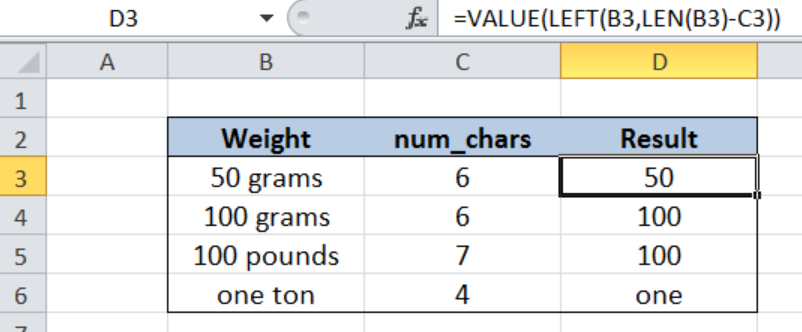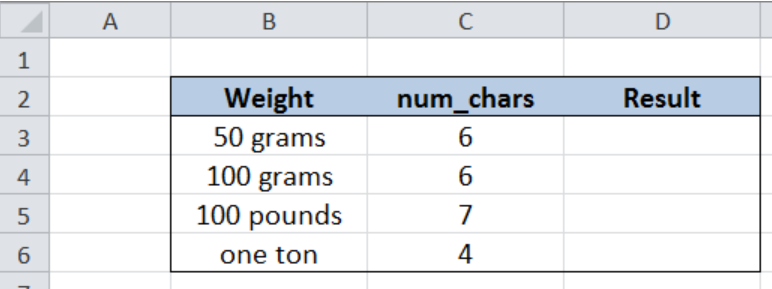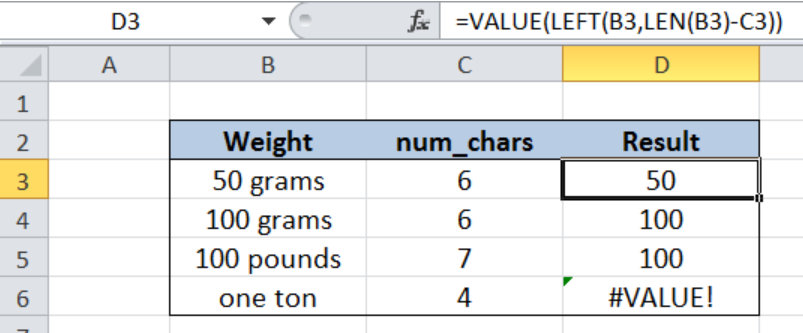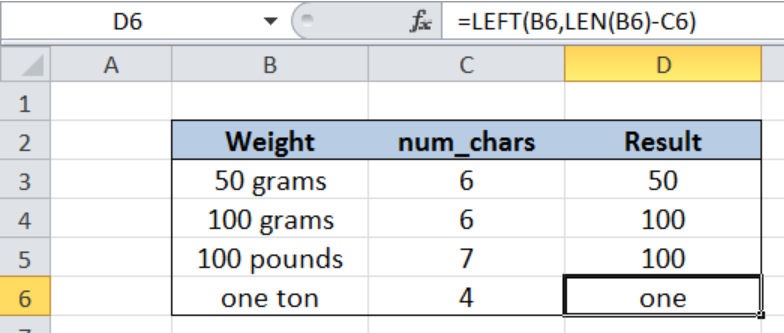Get instant live expert help with Excel or Google Sheets“My Excelchat expert helped me in less than 20 minutes, saving me what would have been 5 hours of work!”

#### Post your problem and you’ll get Expert help in seconds.

Your message must be at least 40 characters
Our professional Expert are available now. Your privacy is guaranteed.

# Remove characters from right

While working with Excel, we are able to retrieve only the specific parts of a text string that we want by using the VALUE, LEFT and LEN  functions.  This step by step tutorial will assist all levels of Excel users in removing characters from the right side of a cell or text string.Figure 1. Final result: Remove characters from the right

Formula 1: `=VALUE(LEFT(B3,LEN(B3)-C3)) `

Formula 2:  `=LEFT(B6,LEN(B6)-C6) `

## Syntax of the VALUE Function

`=VALUE(text)`

• text – a text that we want to convert to a numeric value; text can either be enclosed in quotation marks or a cell reference
• text can be in time or date format, or a constant number; if text is in a format other than these three, VALUE function returns the error value “#VALUE!

## Syntax of the LEFT function

LEFT extracts a substring from the left side of a text string

`=LEFT (text,[num_chars])`

• text – the text string containing the characters or substring we want to extract
• num_chars   Optional; determines the number of characters we want to extract

## Syntax of the LEN function

LEN returns the length of a text string in number of characters

`=LEN(text)`

• text  – the text whose length we want to determine. Spaces are counted as characters.

## Setting up Our Data

Our table contains a list of Weights (column B) and num_chars (column C).  Num_chars represents the number of characters that we want to remove from the right side of the values in column B.  The resulting substring will be recorded in column D under “Result”.Figure 2. Sample data to remove characters from right of a cell

## Remove characters from right of cell

We only want specific parts of the weight values in column B.  In order to obtain only the numeric values and remove succeeding characters from the right, we use the VALUE, LEFT and LEN functions.  Let us follow these steps:

Step 1.  Select cell D3

Step 2.  Enter the formula: `=``VALUE(LEFT(B3,LEN(B3)-C3))`

Step 3:  Press ENTER

Step 4:  Copy the formula in cell D3 to cells D4:D6 by clicking the “+” icon at the bottom-right corner of cell D3 and dragging it down

Our formula extracts a substring from the left side of the text in B3, hence removing characters from the right.  The number of characters we want to extract is determined by the LEN function `LEN(B3)-C3`.  The length of B3 “50 grams” is 8 characters including the space, and C3 is 6.  Hence, the number of characters we want to extract from the left side is (8 – 6) or 2.

As a result, six characters are removed from the right of B3, leaving the two characters “50”.  The VALUE function converts the resulting text into a numeric value.

Copying the formula to cells D4 and D5 returns the substrings we want as shown below.Figure 3. Remove characters from right using VALUE, LEFT and LEN

Note, however, that our formula returns an error value when the resulting text has no equivalent numeric value, as shown in cell D6.

Note: The VALUE function is only necessary when we want to obtain the numeric values of the resulting text string.

The work-around, therefore, for the last example is by not using the VALUE function.  Let us enter this formula in cell D6:

`=LEFT(B6,LEN(B6)-C6)`

Our formula removes four characters from the right of cell B6 and  obtains the substring “one”.Figure 4. Output: Remove characters from right using LEFT and LEN

Most of the time, the problem you will need to solve will be more complex than a simple application of a formula or function. If you want to save hours of research and frustration, try our live Excelchat service! Our Excel Experts are available 24/7 to answer any Excel question you may have. We guarantee a connection within 30 seconds and a customized solution within 20 minutes.

### Did this post not answer your question? Get a solution from connecting with the expert.Another blog reader asked this question today on Excelchat:
Solution examplesI have text and number in multiple cells (Ex: Cell 1: 4S, Cell 2: 3.5A, Cell 3: 8O) and want to sum the number values only (Ex: 4 + 3.5 + 8). How can I do this?
Solved by O. A. in 60 minsI need a formula that has these 2 rules. If letter was rec'd between 1st and 15th = 1st of the month following letter rec'd date. If letter was rec'd between 16thst and end of the month= 1st of the 2nd month following letter rec'd date.
Solved by V. F. in 20 minsI need to create a formula that extracts the prefix from column A
Solved by I. Q. in 42 minsI need a column to total (Hours)(85) + (Travel)(.55) per line
Solved by F. Q. in 20 minsTrying to figure out what is wrong with my formula in Oct-ZD tab in column AY and BG.
Solved by O. Q. in 8 mins## Subscribe to Excelchat.coAnother blog reader asked this question today on Excelchat: